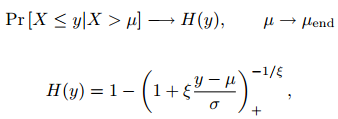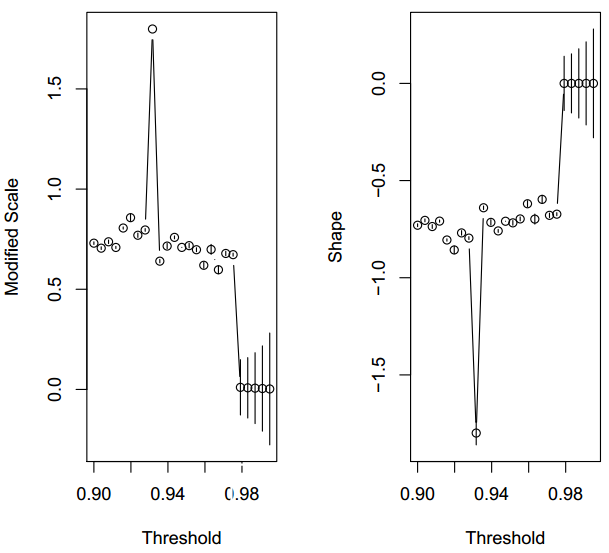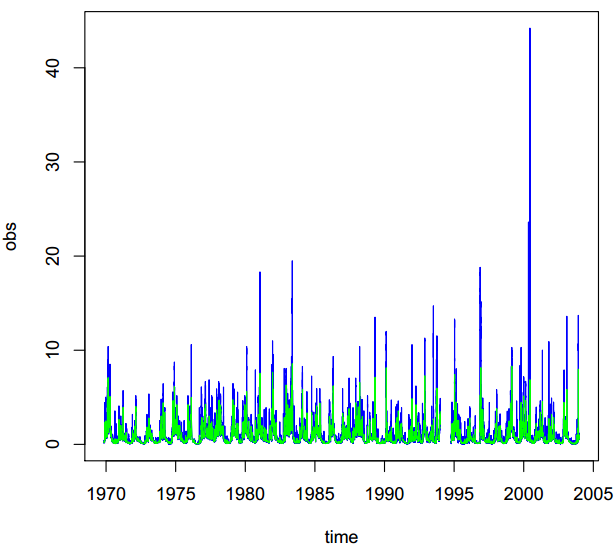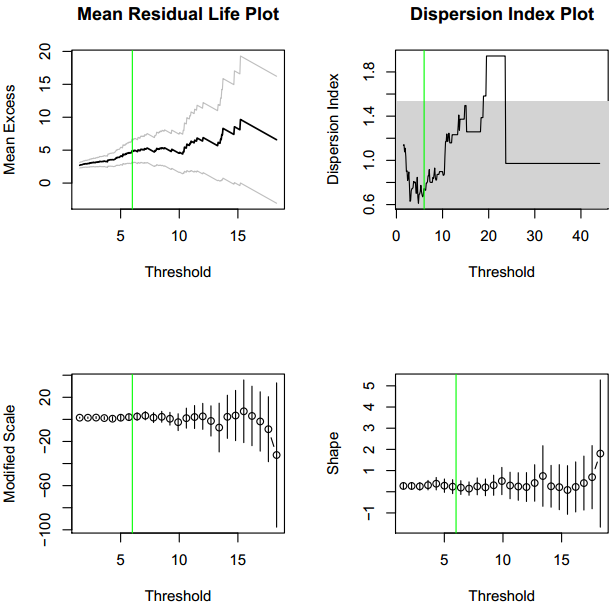# EVT的介绍

## 单变量情况R语言极值理论EVT：基于GPD模型的火灾损失分布分析

## 基本用法随机数和分布函数

``````> rgpd(5, loc = 1, scale = 2, shape = -0.2)
 1.523393 2.946398 2.517602 1.199393 2.541937
> rgpd(6, c(1, -5), 2, -0.2)
 1.3336965 -4.6504749 3.1366697 -0.9330325 3.5152161 -4.4851408
> rgpd(6, 0, c(2, 3), 0)
 3.1139689 6.5900384 0.1886106 0.9797699 3.2638614 5.4755026
> pgpd(c(9, 15, 20), 1, 2, 0.25)
 0.9375000 0.9825149 0.9922927
> qgpd(c(0.25, 0.5, 0.75), 1, 2, 0)
 1.575364 2.386294 3.772589
> dgpd(c(9, 15, 20), 1, 2, 0.25)
 0.015625000 0.003179117 0.001141829``````

## 阈值选择图

``````> x <- runif(10000)
> par(mfrow = c(1, 2))``````可以将置信区间添加到该图，因为经验均值可以被认为是正态分布的（中心极限定理）。但是，对于高阈值，正态性不再成立，此外，通过构造，该图始终会收敛到点（xmax; 0）。

# R语言极值理论 EVT、POT超阈值、GARCH 模型分析股票指数VaR、条件CVaR：多元化投资组合预测风险测度分析

``````> x <- rnorm(10000)
plot(x, u.range = c(1, quantile(x, probs = 0.995)), col = ``````

### L-矩图

L-矩是概率分布和数据样本的摘要统计量。它们类似于普通矩{它们提供位置，离散度，偏度，峰度以及概率分布或数据样本形状的其他方面的度量值{但是是从有序数据值的线性组合中计算出来的（因此有前缀L）。

# 这是一个简单的例子。

``````> x <- c(1 - abs(rnorm(200, 0, 0.2)), rgpd(100, 1, 2, 0.25))
``````

### 色散指数图

``````5, clust.max = TRUE)
> diplot(events, u.range = c(2, 20))``````

# 拟合GPD

## 单变量情况

MLE是一种特殊情况，因为它是唯一允许变化阈值的情况。

``````
scale shape
mom 1.9538076495 0.2423393
mle 2.0345084386 0.2053905
pwmu 2.0517348996 0.2043644
pwmb 2.0624399910 0.2002131
pickands 2.3693985422 -0.0708419
med 2.2194363549 0.1537701
mdpd 2.0732577511 0.1809110
mple 2.0499646631 0.1960452

MLE，MPLE和MGF估计允许比例或形状参数。例如，如果我们要拟合指数分布：

``````
> fit(x, thresh = 1, shape = 0, est = "mle")
Estimator: MLE
Deviance: 322.686
AIC: 324.686
Varying Threshold: FALSE

Threshold Call: 1
Number Above: 100
Proportion Above: 1
Estimates
scale
1.847
Standard Error Type: observed
Standard Errors
scale
0.1847
Asymptotic Variance Covariance
scale
scale 0.03410
Optimization Information
Convergence: successful
Function Evaluations: 7
> fitgpd(x, thresh = 1, scale = 2, est = "mle")
Estimator: MLE
Deviance: 323.3049
AIC: 325.3049
Varying Threshold: FALSE
Threshold Call: 1
Number Above: 100
Proportion Above: 1
Estimates
shape
0.0003398
Standard Error Type: observed
Standard Errors
shape
0.06685
Asymptotic Variance Covariance
shape
shape 0.004469
Optimization Information
Convergence: successful
Function Evaluations: 5
If now, we want to fit a GPD with a varying threshold, just do:
> x <- rgpd(500, 1:2, 0.3, 0.01)
> fitgpd(x, 1:2, est = "mle")
Estimator: MLE
Deviance: -176.1669
AIC: -172.1669
Varying Threshold: TRUE
Threshold Call: 1:2
Number Above: 500
Proportion Above: 1
Estimates
scale shape
0.3261 -0.0556
Standard Error Type: observed
Standard Errors
scale shape
0.02098 0.04632
Asymptotic Variance Covariance
scale shape
scale 0.0004401 -0.0007338
shape -0.0007338 0.0021451
Optimization Information
Convergence: successful
Function Evaluations: 62
``````Asymptotic Variance Covariance
scale1 shape1 scale2 shape2 alpha
scale1 4.602e-03 -2.285e-03 1.520e-06 -1.145e-06 -3.074e-11
shape1 -2.285e-03 2.812e-03 -1.337e-07 4.294e-07 -1.843e-11
scale2 1.520e-06 -1.337e-07 8.956e-04 -9.319e-04 8.209e-12
shape2 -1.145e-06 4.294e-07 -9.319e-04 1.382e-03 5.203e-12
alpha -3.074e-11 -1.843e-11 8.209e-12 5.203e-12 4.003e-12
Optimization Information
Convergence: successful
Function Evaluations: 150

# 拟合双变量POT。所有这些模型均使用最大似然估计量进行拟合。

## 双变量情况

``````vgpd(cbind(x, y), c(0, 2), model = "log")
> Mlog
Estimator: MLE
Dependence Model and Strenght:
Model : Logistic
lim_u Pr[ X_1 > u | X_2 > u] = NA
Deviance: 1313.260
AIC: 1323.260
Marginal Threshold: 0 2
Marginal Number Above: 500 500
Marginal Proportion Above: 1 1
Joint Number Above: 500
Joint Proportion Above: 1
Number of events such as {Y1 > u1} U {Y2 > u2}: 500
Estimates
scale1 shape1 scale2 shape2 alpha
0.9814 0.2357 0.5294 -0.2835 0.9993
Standard Errors``````

``````vgpd(cbind(x, y), c(0, 2), model = "log"
Dependence Model and Strenght:
Model : Logistic
lim_u Pr[ X_1 > u | X_2 > u] = NA
Deviance: 1312.842
AIC: 1320.842
Marginal Threshold: 0 2
Marginal Number Above: 500 500
Marginal Proportion Above: 1 1
Joint Number Above: 500
Joint Proportion Above: 1
Number of events such as {Y1 > u1} U {Y2 > u2}: 500
Estimates
scale1 shape1 scale2 shape2
0.9972 0.2453 0.5252 -0.2857
Standard Errors
scale1 shape1 scale2 shape2
0.07058 0.05595 0.02903 0.03490
Asymptotic Variance Covariance``````
``````scale1 shape1 scale2 shape2
scale1 4.982e-03 -2.512e-03 -3.004e-13 3.544e-13
shape1 -2.512e-03 3.130e-03 1.961e-13 -2.239e-13
scale2 -3.004e-13 1.961e-13 8.427e-04 -8.542e-04
shape2 3.544e-13 -2.239e-13 -8.542e-04 1.218e-03
Optimization Information
Convergence: successful
Function Evaluations: 35

``> pickdep(Mlog)``

# 使用马尔可夫链对依赖关系结构进行建模

对于我们的应用程序，我们模拟具有极值依赖结构的一阶马尔可夫链。

``````mcgpd(mc, 2, "log")
Estimator: MLE
Dependence Model and Strenght:
Model : Logistic
lim_u Pr[ X_1 > u | X_2 > u] = NA
Deviance: 1334.847
AIC: 1340.847
Threshold Call:
Number Above: 998
Proportion Above: 1
Estimates
scale shape alpha
0.8723 0.1400 0.5227
Standard Errors
scale shape alpha
0.08291 0.04730 0.02345
Asymptotic Variance Covariance
scale shape alpha
scale 0.0068737 -0.0016808 -0.0009066
shape -0.0016808 0.0022369 -0.0004412
alpha -0.0009066 -0.0004412 0.0005501
Optimization Information
Convergence: successful
Function Evaluations: 70

### 置信区间

``````
conf.inf.scale conf.sup.scale
2.110881 2.939282

conf.inf.scale conf.sup.scale
1.633362 3.126838

conf.inf.scale conf.sup.scale
2.138851 3.074945

conf.inf.scale conf.sup.scale
2.149123 3.089090``````

``````conf.inf conf.sup
2.141919 NA``````
``````conf.inf conf.sup
0.05757576 0.26363636``````

``````conf.inf conf.sup
2.141919 NA``````
``````conf.inf conf.sup
0.05757576 0.26363636``````
`````` conf.inf conf.sup
8.64712 12.22558``````
``````conf.inf conf.sup
8.944444 12.833333``````
`````` conf.inf conf.sup
8.64712 12.22558``````
``````conf.inf conf.sup
8.944444 12.833333``````

### 模型检查

`` > plot(fitted, npy = 1)``

# 聚类技术

1.第一次超出会启动第一个集群；
2.在阈值以下的第一个观察结果将“终止集群”，除非tim.cond不成立；
3.下一个超过tim.cond的集群将启动新集群；
4.根据需要进行迭代。

`` clu(dat, u = 2, tim.cond = 8/365)``

# 其他

### 返回周期

``````npy retper prob
1 1.8 50 0.9888889
npy retper prob
1 2.2 1.136364 0.6``````

# 河流阈值分析

### 时间序列的移动平均窗口

`` > plot(dat, type = "l", col = "blue")> lines(tsd, col = "green")````````> par(mfrow = c(2, 2))
> plot(even[, "obs"])
> abline(v = 6, col = "green"````````````> events1 <-365, clust.max = TRUE)
> np
time obs
Min. :1970 Min. : 0.022
1st Qu.:1981 1st Qu.: 0.236
Median :1991 Median : 0.542
Mean :1989 Mean : 1.024
3rd Qu.:1997 3rd Qu.: 1.230
Max. :2004 Max. :44.200
NA's : 1.000``````

``> rp2pnpy retper prob1 1.707897 100 0.9941448> scale36.44331``

``conf.inf conf.sup25.56533 90.76633``

``> maxEvent 44.2 shape0.9974115> probnpy retper prob1 1.707897 226.1982 0.9974115``

``conf.inf conf.sup25.56533 90.76633``

Kaizong Ye拓端研究室（TRL）的研究员。

​非常感谢您阅读本文，如需帮助请联系我们！QQ在线咨询

15121130882

0571-63341498

## 关注有关新文章的微信公众号

This will close in 0 seconds